## Generate Conclusions

To use this option, there must be a current data file.

The rule conclusions can be generated, using a method inspired from the fpa (Fast Prototype) algorithm, described in [6,7], or a least squares minimization procedure.

fpa is a simple efficient technique that initializes or updates rule conclusions using data. The ols least squares minimization procedure minimizes the sum of squares of errors (error=difference between inferred output and observed output).

For both options, the output vocabulary can be reduced.

FPA

The rule conclusions are calculated using the observed values of a subset of examples, chosen among the whole data set. This subset, called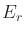for the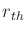rule, is selected for each rule, in function of several criteria that will be explained at the end of this section. First we detail how the conclusion values are calculated, depending on the output type.

In the classification case (class output), the rule conclusion is simply taken as the majority class in.

In the regression case (continuous output), each rule conclusion initialization is done by summing the observed outputs, and weighting them with their corresponding matching degree, as follows. Let us call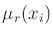the matching degree of the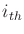example for therule, and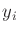the observed output for theexample.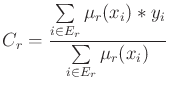(1)

Fuzzy output initialization is done in two steps.

1. calculate a crisp value as for a continuous output (equation 1),
2. set the rule conclusion as the fuzzy set for which the membership degree of the above crisp value is the greatest.

Choice of the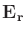subset

Its elements are selected in function of the item matching degree for the rule. The selection can be done in two different ways, depending of the chosen strategy.

The first strategy is called decrease. It retains the examples which most activate the rule. The user can specify two parameters : the cardinality threshold cardmin, and the matching degree matchmin threshold. If the number of examples matching the rule to a degree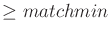is lower than cardmin, the required matching degree is decreased according to a given step (set by the STEP_DEC constant, in the C++ library, whose default value is equal to 0.1). The default matching degree is set to 0.7 by the START_DEC constant. The decrease procedure stops as soon as the required cardinality is reached, or when the required matching degree goes below a limit value MuMin.

This strategy privileges the rule prototypes (in a wide sense), whose definition is given in the glossary (section IV). It is assumed that the examples with a lower matching degree will be dealt with by means of interpolating during the inference procedure.

The other strategy is called minimum. it retains all the examples whose matching degree for the rule isthreshold.

Independently of the applied strategy, a rule will be eliminated if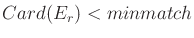, in reason of its insufficient representativity in the data set.

The parameters minmatch and mincard do not play the same role in the two different strategies. The decrease strategy is driven by the mincard parameter, the minmatch parameter being only used as a limit, whereas the minimum strategy is driven by the minmatch parameter, the mincard parameter being only used for checking.

Least square minimization

No parameters for this option.

Reducing the output vocabulary

In the case of a crisp regression output, the rule conclusion values are all different from each other. Reducing the output vocabulary improves the readability of the rule base.

Two choices are available. With the first one (default one), a clustering is performed using the rule conclusions, with the second one it is done by using the data file output values. The clustered values are chosen as the new rule conclusions.

The number of distinct conclusions can be set, or the tolerated loss of performance. Indeed reducing the voabulary usually goes with a loss of accuracy.

Once the vocabulary has been reduced, the output can be fuzzified. This option is available in the Output window, by checking the Fuzzy output box. A standardized fuzzy partition is then built using the rule conclusions as MF centers.